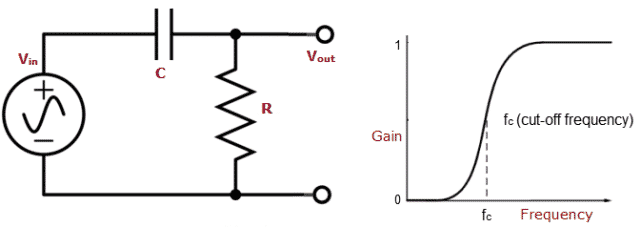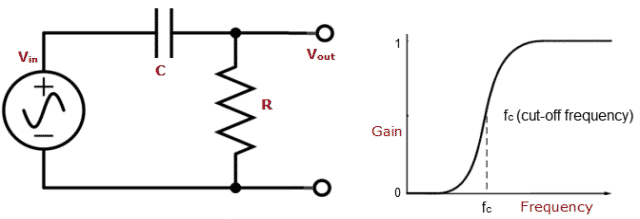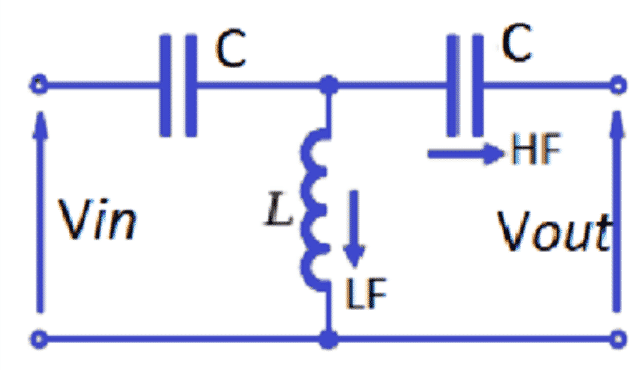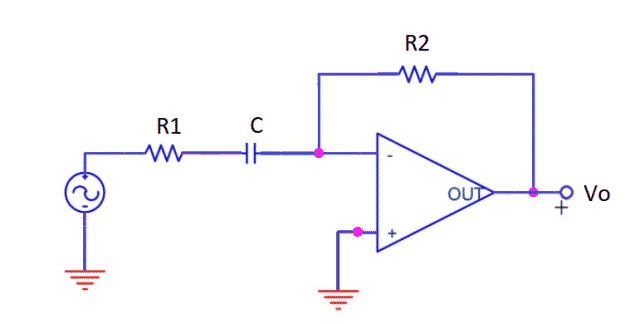Saturday, June 10, 2023

High Pass Filter plays an important role in achieving best sound quality. Some of the circuits have electrical filters which perform signal processing by emphasizing signals in certain ranges and rejecting signals in other frequency ranges out of a mix of different frequencies. One of such filters is High Pass Filter. This post will give you a brief idea about what is High Pass Filter, brief about major types, characteristics, applications, advantages and disadvantages.

## What is High Pass Filter

High Pass (HP) Filter allows certain signals whose frequencies are higher than cut-off frequencies by attenuating signals of lower frequencies. The cut-off frequency value depends on the design of the filter. A series of capacitor and resistor are connected in a basic HP Filter. The capacitor receives the input signal while the output is drawn across the resistor.Fig. 1 – Introduction to High Pass Filter Circuit

The filter circuit can also be built using 2 main components, inductor and capacitor. An inductor allows DC and blocks AC and a capacitor allows AC and blocks DC.

If a signal passes through a capacitor or has grounding path through an inductor then the filter is less attenuated to high frequency signals. This is the reason they are called High Pass Filters. Fig. 1 shows basic High-Pass-Filter Circuit. In this circuit, the capacitor has high reactance to low frequency input signals until cut-off frequency is reached.Fig. 2 – Basic High Pass Filter Circuit

## Types of High Pass Filters

The various types of High Pass Filters include:

• High Pass R-C Filter Circuit
• High Pass R-L Filter Circuit
• Inverted L type High-Pass-Filter Circuit
• T- Type High-Pass-Filter
• π Type High-Pass-Filter
• High-Pass-Filter using Op-Amp
• Butterworth High-Pass-Filter

### High Pass R-C Filter Circuit

In this circuit, the output voltage is measured across the resistor (R). The reactance of the capacitor decreases when frequency increases and therefore the output and gain increases. It is represented as,The capacitor provides significant amount of reactance at low frequencies and hence it blocks them. But, at high frequencies it provides little reactance and allows them to pass through it. At very high frequencies the reactance becomes very small such that the output is almost equal to input and gain is equal to unity.

As the circuit blocks low frequencies and allows high frequency to pass through it, we call it as R-C Circuit. In this circuit the output voltage is measured across the resistor (R). The reactance of capacitor decreases when frequency increases and therefore the output and gain increases. It is represented as,The capacitor provides significant amount of reactance at low frequencies and hence it blocks them. But, at high frequencies it provides little reactance and allows them to pass through it. At very high frequencies the reactance becomes very small such that the output is almost equal to input and gain is equal to unity. As the circuit blocks low frequencies and allows high frequency to pass through it, we call it as R-C Circuit.

The formula to calculate the frequency of RC circuit isLet us consider a R-C High-Pass-Filter which is built with generator, a 10nF Ceramic Capacitor and a 10KΩ Resistor. Now let us calculate the frequency based on the above circuit example.

Hence, the RC circuit will pass the frequencies above 15,923 Hz. Frequencies below 15,923 Hz will be attenuated.

If the input is AC signal into the circuit and place the signal to low frequency like 100 Hz, then the capacitor will block the voltage signal and it will not go through the output. If we increase the frequency to about kHz, then the signal will go through unimpeded. All the high frequency signals pass through without any attenuation.Fig. 3 – Example for R-C Filter Circuit and Characteristics

### High Pass R-L Filter Circuit

In this circuit the resistance (R) provides fixed opposition. The R-L circuit passes through the high frequency signals. An inductor, like capacitor is a reactive device. Inductors pass low frequency signals with little resistance, while it provides great resistance to signals of high frequency. In a simple way, the reactance of inductor L is directly proportional to the frequency.

The cut-off frequency for R-L filter circuit is given below.Let us consider that R-L circuit is built with a function generator, a Resistor of 10KΩ, and  Inductor of 470mH. Let us apply the formula and find out the frequency.

This means frequencies above 3,388 Hz will be passed through without attenuation, while below this will get attenuated. The circuit passes the high frequency signals to output, while it filters the low frequency signals through Inductor.Fig. 4 – Example of R-L Filter Circuit

### Inverted L type High Pass Filter Circuit

The reactance of the Capacitor (C) is large and Inductor (L) is small during low frequency and hence output voltage Vout across inductor is very low. Thus, low frequencies are blocked and high frequencies are allowed.Fig. 5 – Example of Inverted L Type Filter Circuit

### T- Type High Pass Filter

In this type of HP Filter, additional capacitor is added to improve the filtering action. At low frequency the reactance of capacitor is large and inductor is low. Additional capacitor also provides large reactance and thus at high frequencies XL becomes large and XC is reduced such that output voltage is developed across L.Fig. 6 – Example of T- Type Filter Circuit

### π Type High pass Filter

In this type of filter, two inductors are grounded in parallel and one capacitor is connected in series between input and output. The inductors filter the low frequencies and output voltage is taken across the inductors.Fig. 7 – Example of π Type Filter Circuit

### High Pass Filter using Op-Amp

It is also called as Active HP filter because an active element Op-Amp is used in the circuit along with the passive element capacitor and resistor. Op-Amp controls the cutoff frequency and output response of the filter circuit. The cut off frequency is defined by bandwidth and gain characteristics of Op-amp. Hence this filter acts as Band Pass Filter.Fig. 8 – Example of High Pass Filter using Op-Amp

### Butterworth High Pass Filter

Butterworth Filter was first described by physicist Stephen Butterworth in the paper “On the Theory of Filter Amplifiers”. Butterworth stated that: “An ideal filter should reject the unwanted frequencies and should have uniform sensitivity of the required frequencies”. It is a low pass filter but by modification HP Filter can be designed.

For first order High-Pass-Filter, Unit Gain is H(jω) = jω/(jω+ ω_c)

For n such series of filters which upon solving equals to;n controls the order of transition. Higher the order, rapid the transition so at n=∞ Butterworth filter becomes an ideal High-Pass-Filter.

## Applications of High Pass Filter

The applications of HP Filter are:

• Used in speakers for amplification.
• It is used in image processing for sharpening the images.
• It prevents amplification of DC current which can harm amplifiers.
• They are applied for AC coupling.
• They are used in various control systems, audio processing.

## Advantages of High Pass Filter

The advantages of HP Filter are:

• They are used in audio processing, which filters unwanted noise.
• Butterworth filter has the magnitude response zero at the geometric center of the passband. It has the simple transfer function where the coefficients of the polynomials are easy to calculate.
• They are used in various applications such as broadcast receivers to select desired channel frequency.

## Disadvantages of High Pass Filter

The disadvantages of HP Filter are:

• If component value is not selected correctly then we end up filtering the frequency which we actually need.
• They filter out the DC offset of a signal.
• When using active filters, if we don’t select correct components then we get unwanted ripples in the passband or the stopband, or unwanted phase shifts in certain frequencies.
```Also Read:
Filters - Classification, Characteristics, Types, Applications & Advantages
Waveguide - Classification, Modes, How it Works, Application, Advantage
How to Make Simple Inverter at Home - Step by Step
```Madhuri is a B.E (Computer science) and has experience in IBM as Software engineer. She is an Author, Editor and Partner at Electricalfundablog.com.
RELATED ARTICLES